# Introducing Excel XP Microsoft Office Excel 2007 or

• Slides: 11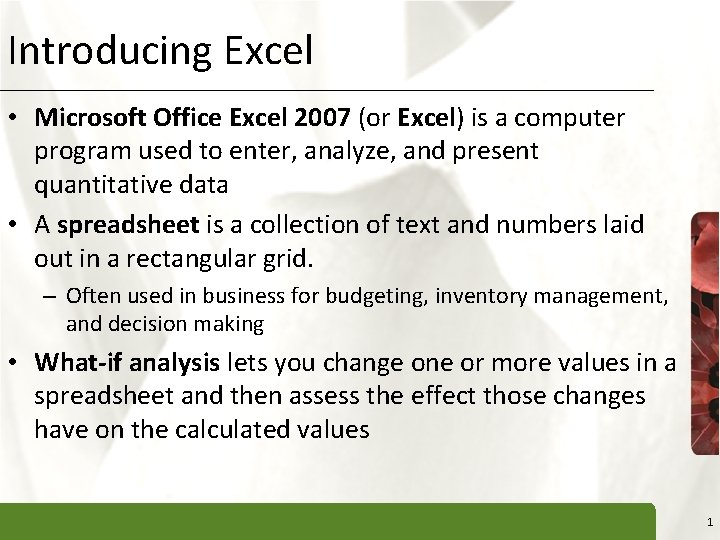Introducing Excel XP • Microsoft Office Excel 2007 (or Excel) is a computer program used to enter, analyze, and present quantitative data • A spreadsheet is a collection of text and numbers laid out in a rectangular grid. – Often used in business for budgeting, inventory management, and decision making • What-if analysis lets you change one or more values in a spreadsheet and then assess the effect those changes have on the calculated values 1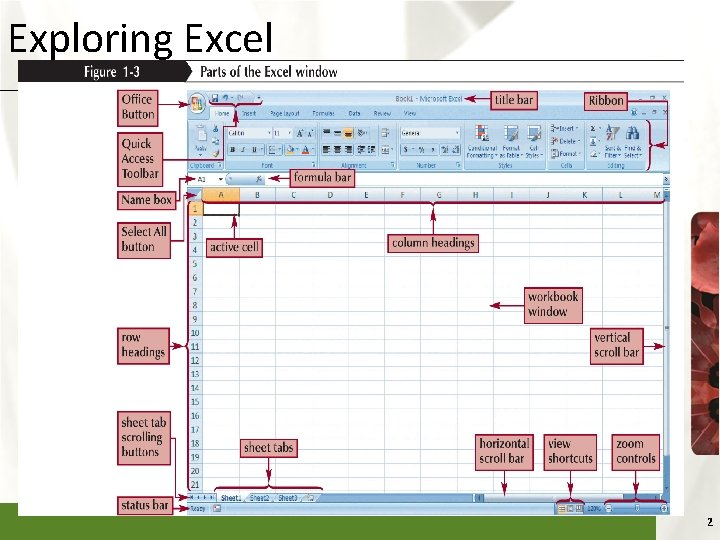Exploring Excel XP 2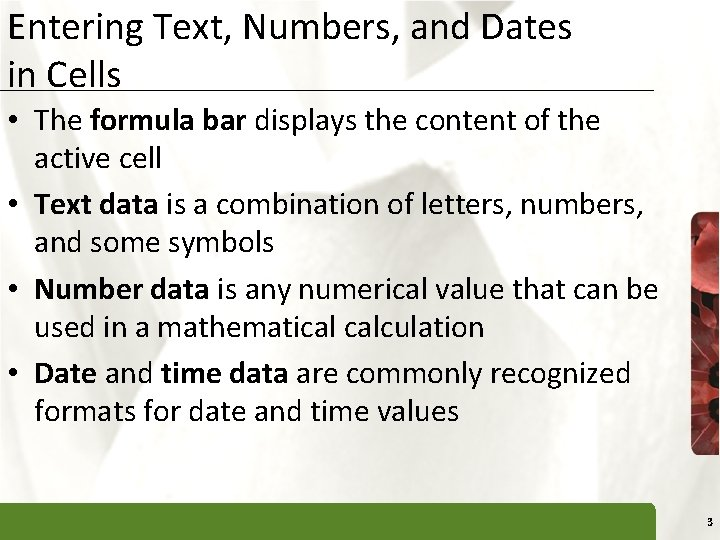Entering Text, Numbers, and Dates in Cells XP • The formula bar displays the content of the active cell • Text data is a combination of letters, numbers, and some symbols • Number data is any numerical value that can be used in a mathematical calculation • Date and time data are commonly recognized formats for date and time values 3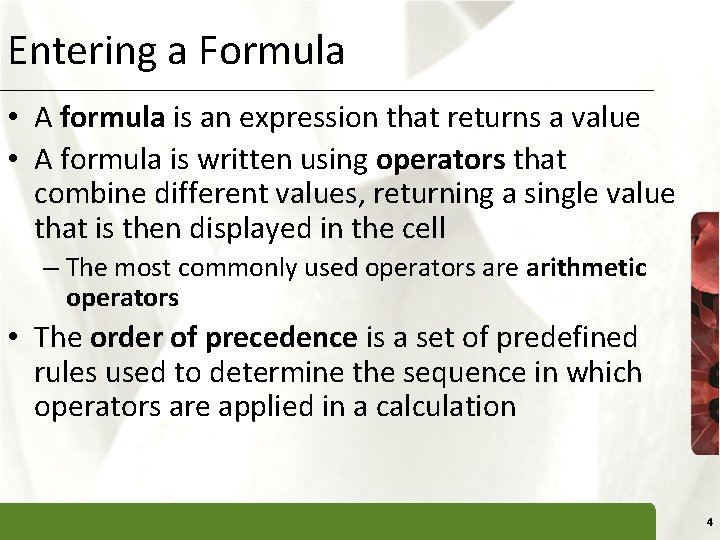Entering a Formula XP • A formula is an expression that returns a value • A formula is written using operators that combine different values, returning a single value that is then displayed in the cell – The most commonly used operators are arithmetic operators • The order of precedence is a set of predefined rules used to determine the sequence in which operators are applied in a calculation 4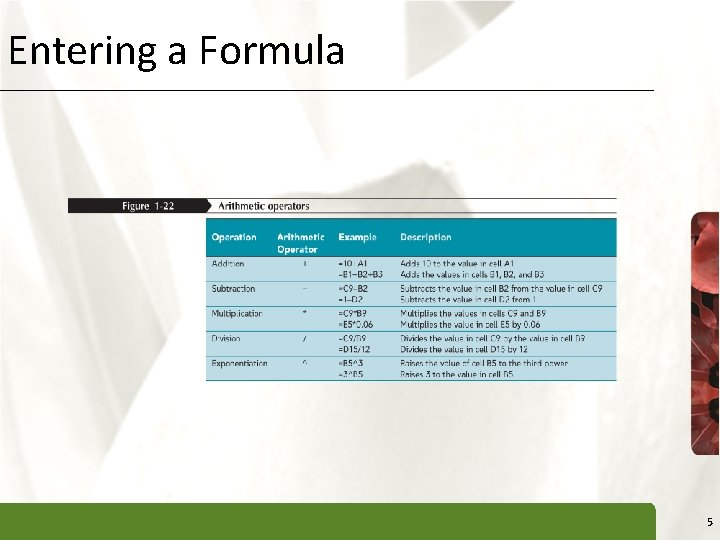Entering a Formula XP 5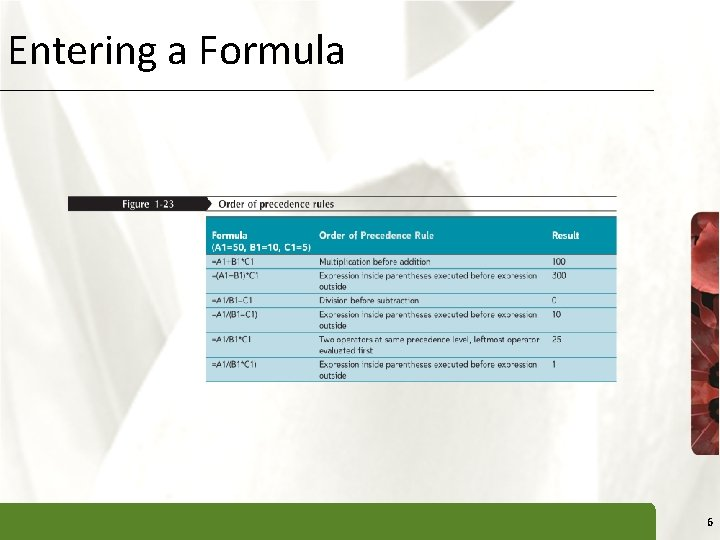Entering a Formula XP 6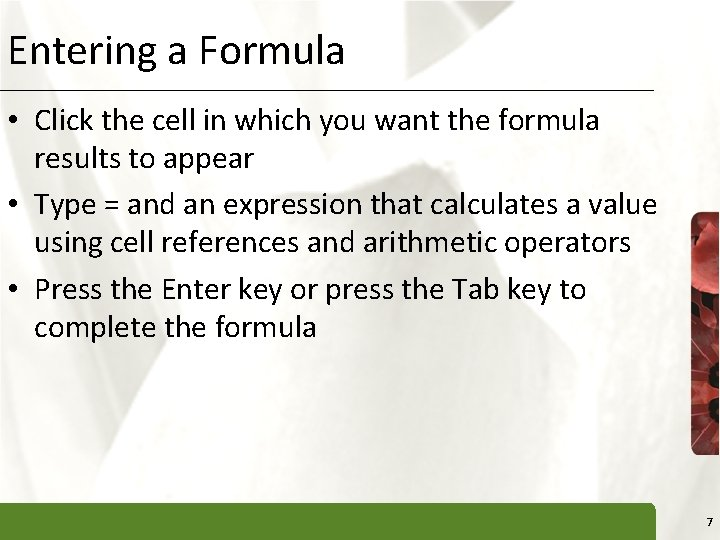Entering a Formula XP • Click the cell in which you want the formula results to appear • Type = and an expression that calculates a value using cell references and arithmetic operators • Press the Enter key or press the Tab key to complete the formula 7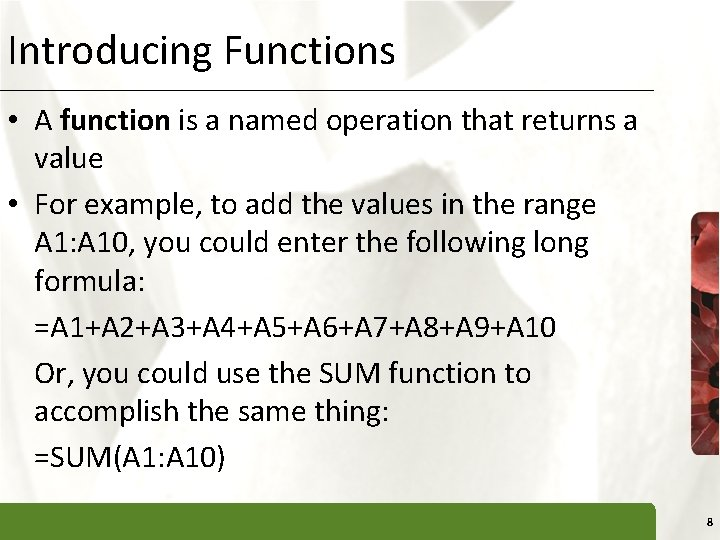Introducing Functions XP • A function is a named operation that returns a value • For example, to add the values in the range A 1: A 10, you could enter the following long formula: =A 1+A 2+A 3+A 4+A 5+A 6+A 7+A 8+A 9+A 10 Or, you could use the SUM function to accomplish the same thing: =SUM(A 1: A 10) 8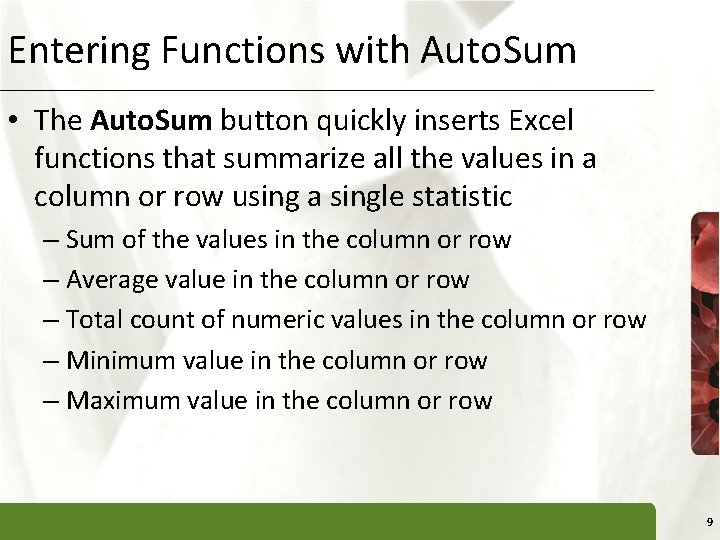Entering Functions with Auto. Sum XP • The Auto. Sum button quickly inserts Excel functions that summarize all the values in a column or row using a single statistic – Sum of the values in the column or row – Average value in the column or row – Total count of numeric values in the column or row – Minimum value in the column or row – Maximum value in the column or row 9Inserting and Deleting a Worksheet XP • To insert a new worksheet into the workbook, right-click a sheet tab, click Insert on the shortcut menu, select a sheet type, and then click the OK button • You can delete a worksheet from a workbook in two ways: – You can right-click the sheet tab of the worksheet you want to delete, and then click Delete on the shortcut menu – You can also click the Delete button arrow in the Cells group on the Home tab, and then click Delete Sheet 10Changing Worksheet Views XP • You can view a worksheet in three ways: – Normal view simply shows the contents of the worksheet – Page Layout view shows how the worksheet will appear on the page or pages sent to the printer – Page Break Preview displays the location of the different page breaks within the worksheet 11